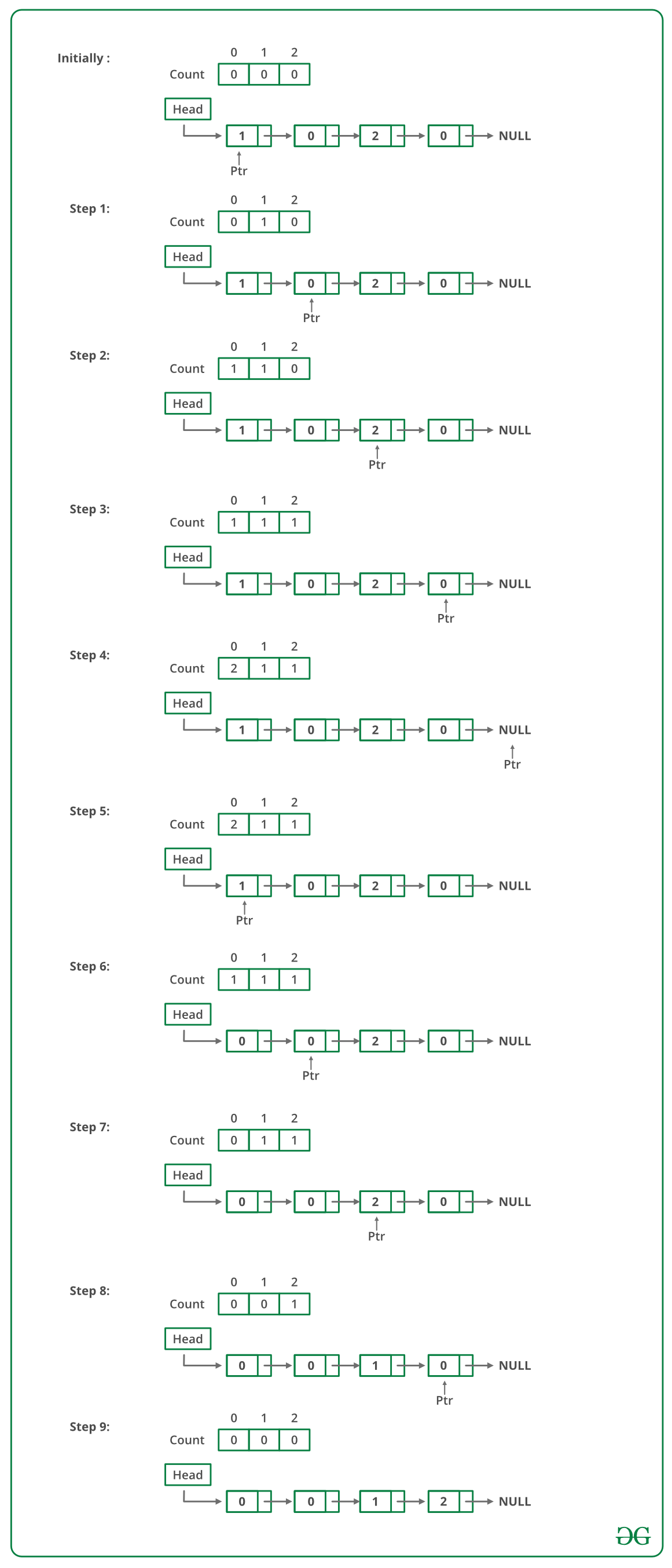Open in App
Not now

# C++ Program For Sorting A Linked List Of 0s, 1s And 2s

• Last Updated : 10 Nov, 2022

Given a linked list of 0s, 1s and 2s, sort it.
Examples:

```Input: 1 -> 1 -> 2 -> 0 -> 2 -> 0 -> 1 -> NULL
Output: 0 -> 0 -> 1 -> 1 -> 1 -> 2 -> 2 -> NULL

Input: 1 -> 1 -> 2 -> 1 -> 0 -> NULL
Output: 0 -> 1 -> 1 -> 1 -> 2 -> NULL```

Source: Microsoft Interview | Set 1

Following steps can be used to sort the given linked list.

• Traverse the list and count the number of 0s, 1s, and 2s. Let the counts be n1, n2, and n3 respectively.
• Traverse the list again, fill the first n1 nodes with 0, then n2 nodes with 1, and finally n3 nodes with 2.

Below image is a dry run of the above approach:Below is the implementation of the above approach:

## C++

 `// C++ Program to sort a linked list ` `// 0s, 1s or 2s ` `#include ` `using` `namespace` `std;`   `// Link list node ` `class` `Node ` `{ ` `    ``public``:` `    ``int` `data; ` `    ``Node* next; ` `}; `   `// Function to sort a linked list ` `// of 0s, 1s and 2s ` `void` `sortList(Node *head) ` `{ ` `    ``// Initialize count of '0', '1' ` `    ``// and '2' as 0 ` `    ``int` `count = {0, 0, 0}; ` `    ``Node *ptr = head; `   `    ``/* Count total number of '0', '1' and '2' ` `       ``count will store total number of '0's ` `       ``count will store total number of '1's ` `       ``count will store total number of '2's */` `    ``while` `(ptr != NULL) ` `    ``{ ` `        ``count[ptr->data] += 1; ` `        ``ptr = ptr->next; ` `    ``} `   `    ``int` `i = 0; ` `    ``ptr = head; `   `    ``/* Let say count = n1, count = n2 ` `       ``and count = n3.` `       ``Now start traversing list from head node, ` `       ``1) fill the list with 0, till n1 > 0 ` `       ``2) fill the list with 1, till n2 > 0 ` `       ``3) fill the list with 2, till n3 > 0 */` `    ``while` `(ptr != NULL) ` `    ``{ ` `        ``if` `(count[i] == 0) ` `            ``++i; ` `        ``else` `        ``{ ` `            ``ptr->data = i; ` `            ``--count[i]; ` `            ``ptr = ptr->next; ` `        ``} ` `    ``} ` `} `   `// Function to push a node` `void` `push (Node** head_ref, ` `           ``int` `new_data) ` `{ ` `    ``// Allocate node ` `    ``Node* new_node = ``new` `Node();`   `    ``// Put in the data ` `    ``new_node->data = new_data; `   `    ``// Link the old list of the ` `    ``// new node ` `    ``new_node->next = (*head_ref); `   `    ``// Move the head to point to the ` `    ``// new node ` `    ``(*head_ref) = new_node; ` `} `   `// Function to print linked list ` `void` `printList(Node *node) ` `{ ` `    ``while` `(node != NULL) ` `    ``{ ` `        ``cout << node->data << ``" "``; ` `        ``node = node->next; ` `    ``} ` `    ``cout << endl; ` `} `   `// Driver code` `int` `main(``void``) ` `{ ` `    ``Node *head = NULL; ` `    ``push(&head, 0); ` `    ``push(&head, 1); ` `    ``push(&head, 0); ` `    ``push(&head, 2); ` `    ``push(&head, 1); ` `    ``push(&head, 1); ` `    ``push(&head, 2); ` `    ``push(&head, 1); ` `    ``push(&head, 2); `   `    ``cout << ``"Linked List Before Sorting"``; ` `    ``printList(head); `   `    ``sortList(head); `   `    ``cout << ``"Linked List After Sorting"``; ` `    ``printList(head); `   `    ``return` `0; ` `} ` `// This code is contributed by rathbhupendra`

Output:

```Linked List Before Sorting
2  1  2  1  1  2  0  1  0